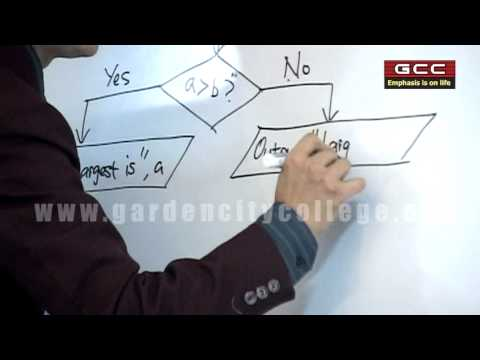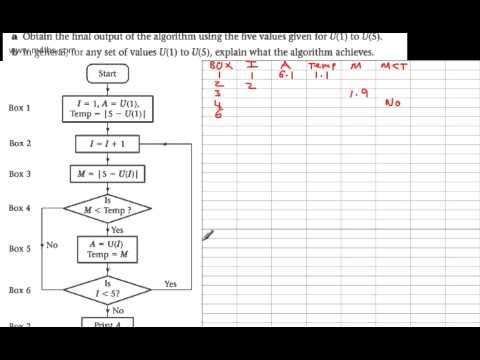## Conversion of algorithm/flowchart

Subject: Computer Basics

#### Overview

Example to convert algorithms and flowchart to high level language C.
##### Conversion of algorithm/flowchart

Write an algorithm to compute the sum of the first 100 positive integers.

1. Set sum to 0.
2. Set x to 1.
3. Repeat while x <= 100:
1. Set sum to sum + x.
2. Set x to x+1.
4. Print sum.#### Solution in C programming language:

Sum = 0;
X = 1;
for(X= 1; X<=100; X==)
{
Sum = Sum + X;
}
print Sum;

##### Things to remember

• It includes every relationship which established among the people.
• There can be more than one community in a society. Community smaller than society.
• It is a network of social relationships which cannot see or touched.
• common interests and common objectives are not necessary for society.
##### Videos for Conversion of algorithm/flowchart##### Concepts of Algorithm, Flow Chart & C Programming##### D1 Algorithms Implementing an algorithm from a flow chart Basic Example

An algorithm is a sequence of steps required to solve a particular problem. The concept of algorithm was given by ALKHOWARISMI.

1. Some characteristic of an algorithm are listed below:
2. Each and every instruction should be precise and unambiguous.
3. An algorithm should have finite number of steps.
4. An algorithm should produce correct result.
5. An algorithm should not use particular programming language.
Flowchart is a graphical representation of program solving steps so that it makes clear about the program. The concept of flowchart was given by John Vonn Neuman 1945.
Types of flowchart are:
a) System Flowchart b) Program Flowchart
The common guidelines (properties) of flowchart are:
The flowchart should have only one start and ending points.
Flow lines shouldn't intersect each other.
Flowchart should not contain the programming language.
The flowchart should be neat and clear for the user.

Some of the symbols used in flowchart along with their meanings are listed below:The step by step solution of a problem in a picture form is known as flowchart.
Algorithm is defined as a set of rules that define how a particular problem can be solved in finite number of steps.
An algorithm and flowchart to compute the sum of the first 100 positive integers.

1. Set x
2. Set sum to 0.
3. to 1.
4. Repeat while x <= 100:
1. Set sum to sum + x.
2. Set x to x+1.
5. Print sum.